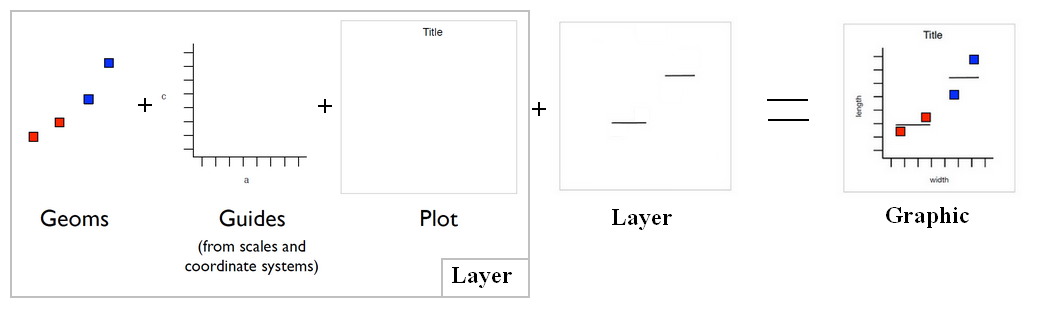# R - NA (Not Available)

NA (Not Available|Missing Values) is a logical constant.

See

````?NA`
```
``````> str(NA)
logi NA```
```

NA values have a class. There are integer NA, character NA, etc.

NA means “Not Available”.

NA is a logical constant of length 1 which contains a missing value indicator.

## Function

### is.na

• Is NA NA ?
``````> is.na(NA)
 TRUE```
```
• Where is NA ?
``````> v =  c(1, NA, 2)
> is.na(v)
 FALSE  TRUE FALSE```
```
``````> is.na(NaN)
 TRUE```
```

## Management

### analytics

````vapply(data.frame, function(x) mean(!is.na(x)), numeric(1))`
```

### remove

#### vector

``````v =  c(1, NA, 2)
> v[!is.na(v)]
 1 2```
```

#### matrix

``````colA = c(1, 2, 3, 4, NA, 6)
colB = c(1, 2, NA, 4, 5, 6)
m=cbind(colA,colB)
m```
```
``````colA colB
[1,]    1    1
[2,]    2    2
[3,]    3   NA
[4,]    4    4
[5,]   NA    5
[6,]    6    6
```
```
``````rowWithoutNa = complete.cases(m)
rowWithoutNa```
```
``````  TRUE  TRUE FALSE  TRUE FALSE  TRUE
```
```
````m[rowWithoutNa,]`
```
``````colA colB
[1,]    1    1
[2,]    2    2
[3,]    4    4
[4,]    6    6
```
```

### eliminate the row

to eliminate any row in the data frame that's got a missing value in it, there's a nice function called na.omit

````myDataFrame=na.omit(myDataFrame)`
```

### Count

in a variable

````with(dataFrame,sum(is.na(myVariableName)))`
```

### Create them

````myNaVector=rep(NA,10)`
```

Recommended PagesGgplot - Color

Colour is an aesthetic (plot parameter) that changes the bar outline color. See also: fill to change the interior colouring. alpha for the transparency A name, e.g., “red”. R has 657 built-in...ORE - Snippet

ore snippet Connect to the database. Load Data Frame to table Create IRIS_TABLE_N that does not contain SPECIES, the nonnumeric column: The sample null.R is the only sample that does...R - (Mathematical|Logical) Operators

& boolean and | boolean for xor exactly or ! not any any true all all true Not (!) Many operations in R are vectorized. < Less than > Greater than == Equal to <= Less than or equal...R - Condition

A condition is a length-one logical vector (FALSE of TRUE) that is not NA. Conditions are always evaluated from left to right.R - Constant

R - ConstantR - Factor (Category, Enumerated Type)

The factor function is used to encode a vector as a factor (ie categorical data). When used with a numeric or a date, a binning function will return a factor. From numeric to a category (For instance,...R - Matrix

The matrix object in R is an array of two dimensions with the same class (data type). You can create a matrix with three methods: the matrix method the columns and rows bindings methods the...R - NaN

NaN in R. It defines an undefined mathematical operations and then is also an NA (Missing values) Undefined mathematical operations Is Na NaN ? Is NaN Na ?R - Vector

The Vector in R contains elements of the same class. A Vector with different class of objects is a list. A vector (as every object) can also have . Almost all data in R is a vector or is based upon...